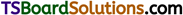# TS Inter 1st Year Chemistry Notes Chapter 5 Stoichiometry

Here students can locate TS Inter 1st Year Chemistry Notes 5th Lesson Stoichiometry to prepare for their exam.

## TS Inter 1st Year Chemistry Notes 5th Lesson Stoichiometry

→ The standard reference for relative atomic masses is Carbon – 12.

→ $$\frac{1}{12}$$th of the mass of C -12 is called atomic 12 mass unit (amu).

→ The value of amu is 1.66 × 10-24 gm. This is known as Avogram.

→ The weight of a substance in grams, numerically equal to its molecular weight is called gram molecular weight or gram mole.

→ Number of atoms present in one gram molecular weight of element is called Avogadro’s number. Its value is 6.023 × 1023.

→ The volume occupied by one gram molecular weight of a gas at STP is called gram molar volume (GMV). Its value is 22.4 lits or 22,400 cc.

→ Mole is the SI unit for the amount of a substance.

→ Mole is that mass of a substance which contains Avogadro number of structural units.

→ The apparent charge that an atom appears to have in its combined state is called its oxidation number. It may be + ve (or) – ve (or) fractional or zero.

→ The element that never exhibits + ve oxidation number is Fluorine.→ The process of removal of electron is called Oxidation.

→ The process of addition of electron is called Reduction.

→ The substance which gains electrons is called Oxidant.

→ The substance which loses electrons is called Reductant.

→ A chemical reaction which involves both oxidation and reduction is called redox re-action.

→ Redox reactions can be balanced by
(a) Ion electron method
(b) Oxidation number method.

→ The actual masses of substances conveyed by a balanced equation is known as Stoichiometry.

→ One hundred times to the weight ratio of an element and the compound containing the element is called percentage composition of the element.

→ The formula that gives the simplest ratio of atoms of the constituent elements present in a compound is called empirical formula.

→ The formula that gives the exact number of atoms of the constituent elements present in a compound is called Molecular formula.

→ Molecular formula = Empirical formula × n
where, n = $$\frac{\text { Molecular formula weight }}{\text { Empirical formula weight }}$$

→ Increase in oxidation number is oxidation and decrease in oxidation number is reduction.